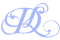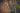# An intro to Functional Programming - #30DaysofFP Week 1

7 min read · August 12, 2019

Category: Web DevelopmentTagged with: Functional Programming#30DaysofFPPhoto by Clint Adair

1.5 weeks ago I set out to learn more about Functional Programming with a 30 day challenge. I started knowing little about functional programming, apart from the various array methods within JavaScript. Kyle Simpson's Functional Light JavaScript book, has been a great introduction of concepts and practical application. For those interested, the book can also be read for free on GitHub.

In this post, I share some of the practical concepts of functional programming I have learned since starting this challenge, as well as refactor a code example using functional composition. There's lots of code examples and functional goodies along the way. Note: The code snippets include several functional programming helper functions. An example implementation of these utilities is included in the snippets, but can also be found in libraries such as Ramda.

## Partial Application

Partial application applies some of the arguments that a function needs to operate, and returns a function that is awaiting the remaining arguments. This allows pre-loading a more generic function to make it a more specific one.

``````const partial = (fn, ...presetArgs) =>
(...laterArgs) =>
fn(...presetArgs, ...laterArgs)

const add = (x, y) => x + y
console.log(add(5, 8)); //13

const add5 = partial(add, 5)
console.log(add5(8)); // 13``````

When we call partial, it gathers all of the arguments after the first one and stores them in the `presetArgs` parameter. This then returns a function which awaits any additional number of arguments. Once those arguments are received, the function is called, spreading `presetArgs` and `laterArgs` into the function call. Using this pattern we can create more specific implementations of a generic function. This could be used for other cases such as preloading a url for a fetch call, while awaiting query parameters or post data, and more. Some additional implementation examples are included in the sections below.

## Currying

Currying is another means of partially applying functions, but is different in it's approach. Unlike the `partial` function described above, currying returns functions that only accept one argument at a time. This can provide additional control over making the functions more specific and reusable.

Before diving into the specific implementations of currying it is important to understand the concept of arity. Arity refers to the number of arguments a function can accept. For example, our `add` function above accepts two arguments and has an arity of 2. A function which accepts three argument has an arity of 3. There are some specific terms to describe an arity of 1 or 2.

• A function which has an arity of 1 is unary.
• A function which has an arity of 2 binary.
• Functions with three or more arguments are referred to as arity of n, i.e. arity of 3

Currying takes a function of any given arity and breaks them into that many unary functions. For example:

``````const curry = (fn, arity = fn.length, nextCurried) =>
(nextCurried = prevArgs =>
nextArg => {
const args = [...prevArgs, nextArg]
if (args.length >= arity) {
return fn(...args)
}
return nextCurried(args)
})([])

const add = (x, y) => x + y
const curriedAdd = curry(add)

const add5 = curriedAdd(5)
console.log(add5(12)); // 17

console.log(curriedAdd(5)(12)); // 17``````

The `curry` utility only accepts the first argument it is provided before returning the new function. For instance, `curriedAdd(5, 3)(12)` would still evaluate to 17 as the 3 is discarded.

## Functional Composition

Functional programming enables arranging functions to work together to complete a larger block of logic. This provides greater code reuse, and can preload the logic of more complex functions, waiting to receive their data.

These concepts already exist with some of the array methods in JavaScript. For example, let's say that I want to write a function which accepts an array of numbers, filters out any that are less than 5, and then returns the sum of the remaining numbers. Writing this function with array methods may look something like this:

``````const nums = [2, 5, 8, 12, 3]

const sum = nums
.filter(num => num > 5)
.reduce((acc, val) => acc + val)

console.log(sum); // 20``````

There is nothing wrong with this approach, and I know I have written many functions in a similar manner. However, the logic for the given filter and addition is locked into this particular implementation. Since filter and reduce both accept functions, we can refactor the logic out of this implementation to their own external functions:

``````const nums = [2, 5, 8, 12, 3]

const greaterThan5 = num => num > 5
const adder = (x, y) => x + y

const sum = nums.filter(greaterThan5).reduce(adder)
console.log(sum); // 20``````

This code snippet is logically the same as the previous implementation. The main difference is that we have pulled out the logic for the functions so that they can be reused in other parts of the codebase. It can also be argued that the callsite of calculating `sum` is clearer. The filter and reduce now focus on what is happening rather than how it is being achieved. This function is now more declarative rather than imperative. If you are unfamiliar with these concepts, I highly recommend reading Tyler McGinnis' breakdown of the them.

This approach still maintains the chaining of the filter and reduce onto an array. Using our partial utility from earlier, we can abstract some of this chaining further to pre-load a filter function that is awaiting an array of data.

``````const nums = [2, 5, 8, 12, 3]

const partial = (fn, ...presetArgs) =>
(...laterArgs) =>
fn(...presetArgs, ...laterArgs)
const filter = (fn, arr) => arr.filter(fn)
const reduce = (fn, arr) => arr.reduce(fn)

const greaterThan5 = num => num > 5
const adder = (x, y) => x + y
const filterGreaterThan5 = partial(filter, greaterThan5)
const reduceAdder = partial(reduce, adder)

const sum = reduceAdder(filterGreaterThan5(nums))
console.log(sum); // 20``````

We are continuing to move farther into the functional programming and composition approach. Here we have created utility helpers for filter and reduce. Both accept a function as the first argument, and the array to operate on as the second argument. Using our `partial` function defined earlier, we can preload these functions with our filter and adder from previous implementations. Here `filterGreaterThan5` is a function which is waiting for an array and then will filter that array with our `greaterThan5` function. Similarly, `reduceAdder` is a function awaiting an array and then will reduce the array with our `adder` function.

In this example the functions called to calculate sum may seem strange. I know it was for me at first. Unlike English, nested functions like this are read inside out. In other words this can be read as follows:

1. `filterGreaterThan5` is given a `nums` argument and executes.
2. The output of `filterGreaterThan5` (another array) is passed in as the input to `reduceAdder`
3. `reduceAdder` runs on this input. The output of this function is assign the to the const `sum`.

This approach uses more functions, but each one is smaller in scope. As we build up our application we create functions more specific to our use case. A major benefit of this approach is that each of the functions can now be used elsewhere in the application without rewriting the logic. One last obstacle and point of refactoring for our example is cleaning up the nested function calls. This particular example only has the calls nested 2 layers deep, but you can imagine that this could become tedious to do consistently. Thankfully there is a `compose` function utility that can help us out.

Compose is a function which will accept other functions as it's arguments, And programmatically wrap them inside each other. The end result is a function waiting for the data. This allows us to not manually wrap our functions as in the above example.

``````const nums = [2, 5, 8, 12, 3]

// Utility functions
const partial = (fn, ...presetArgs) =>
(...laterArgs) =>
fn(...presetArgs, ...laterArgs)
const compose = (...fns) =>
result =>
[...fns].reverse().reduce((result, fn) =>
fn(result),
result)
const filter = (fn, arr) => arr.filter(fn)
const reduce = (fn, arr) => arr.reduce(fn)

const greaterThan5 = num => num > 5
const adder = (x, y) => x + y
const filterGreaterThan5 = partial(filter, greaterThan5)
const reduceAdder = partial(reduce, adder)

const addNumsGreaterThan5 = compose(reduceAdder, filterGreaterThan5)
const sum = addNumsGreaterThan5(nums)
console.log(sum); // 20``````

Our `addNumsGreaterThan5` function now is waiting for an array of numbers. Upon receiving it, the filter and reduce functions will be ran, returning the sum. We can now call this function with any array of numbers within our application. Additionally, this compose structure allows us to swap out on functional LEGO brick for another to change the implementation. For example, we could switch our filter function to one that filters for numbers less than 5 rather than greater than 5.

## Next Steps

Functional programming aims to make code readable and easier to reason about. If functions have direct inputs and outputs, the developer does not need to trace through the functional call stack to see what pieces of the application state are managed. It becomes easier to mentally parse the effects of running the function. Much of this is governed by the purity of the functions and managing side causes and effects, which will be a discussion for next week. Composing smaller functions almakes our code more declarative, focusing on what is happening rather than the implementation details.

Week 1 of my 30 Days of Functional Programming was a wild ride of learning new materials. You can join in the learning discussion on Twitter.

## Related Posts

### 30 Days of Functional Programming

I have long had a fascination with learning new things. However, it is generally unstructured and follows whatever the interest of the moment is. I have been and continue to be a proponent of… Read more